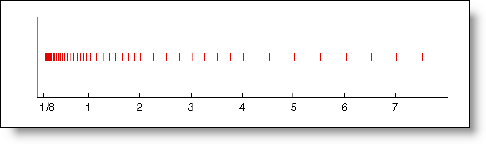# Floating-Point Numbers

Numeric simulation is all about the numbers. In a previous post, I talked about integer and fixed-point number representations. These numbers are especially useful for discrete simulation and embedded systems. For continuous dynamic systems, the values do not represent discrete values but continuously changing functions in time. For this, floating-point numbers provide the flexibility and range of representation needed to store results. In this post, I will review the fundamentals related to floating-point numbers.

Sign, Exponent, Fraction

Floating-point numbers extend the idea of a fixed-point number by defining an exponent. A normalized floating-point number has a sign bit, the exponent, and the fraction.

 sign exponent (e) fraction (f)

The fraction can represent numbers where 0≤X<1. The exponent provides the ability to scale the range of the numbers represented by the fraction. The spacing of floating-point numbers is relative to the number of fractional bits and the magnitude of the number represented. For very large values of the exponent, the spacing between the numbers is large. For small numbers, the spacing is small. This space between the numbers you can represent in floating point is called epsilon, or eps. When calculations result in a number that falls into one of these spaces between floating-point representations, rounding occurs. This rounding introduces an error to the calculation on the order of eps.

Cleve Moler wrote a great article titled Floating Points. It gives a great explanation of how floating point works and some of the historical context for the IEEE double precision standard. In the article, he describes a toy floating-point system consisting of one sign bit, a three-bit exponent, and a three-bit fraction.

 sign exponent (e) fraction (f) +/- +/- 21 20 2-1 2-2 2-3

The exponent can hold integer values between -4 and 3. The fraction holds values of 0 to ⅞ with a ⅛ spacing. The value of a normalized floating-point number is:

x = ± (1 + f ) × 2e

The following graphic from Cleve’s article illustrates the spacing between floating point numbers in this toy system.This is my mental image when I think about floating-point numbers and issues of precision in floating point calculations.

Resources

There are many great sources of knowledge about floating-point numbers on the web and everyone seems to have a favorite reference. My favorite is from Cleve, but here are some more resources to check out for yourself.

Floating Points by Cleve Moler

Where Did All My Decimals Go? by Chuck Allison